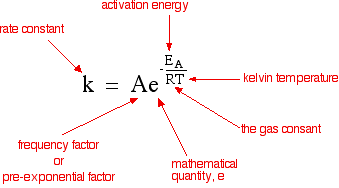Chemguide: Support for CIE A level Chemistry ``` ``` Learning outcome 26: Reaction kinetics 26.1: Simple rate equations, orders of reaction and rate constants ``` ``` Learning outcome 26.1.6 This statement is about the effect of temperature on the rate constant of a reaction and how this affects the rate of the reaction. Before you go on, you should find and read the statement in your copy of the syllabus. You will know from work you have done in in the first half of the course how and why changing the temperature changes the rate of a reaction. If you have forgotten about this, now is the time to revise it. The rate equation for a reaction between A and B will look like this: rate = k [A]a [B]b where k is the rate constant and a and b are the orders of reaction with respect to A and B. The equation tells you what happens to the rate of reaction when you change the concentrations of A and B. However, it doesn't seem to say anything about what happens if you change the temperature or add a catalyst, for example. Those effects are hidden inside the so-called rate constant - which isn't constant if you change anything other than the concentrations! There is an equation which shows how the value of k varies if you change various things - the Arrhenius equation.Notice that the equation includes a temperature term, and also the activation energy of the reaction, which would change if you added a catalyst. The syllabus statement talks about "explain qualitatively . . .", which means that you don't need to do any maths. But if you did do some maths, you would find that increasing T would increase the value of the rate constant, and so increase the reaction rate. Similarly decreasing the activation energy would also increase the value of the rate constant and again increase the reaction rate. Summary Increasing temperature increases the value of the rate constant, and therefore the rate of the reaction increases. ``` ``` If you are mathematically inclined, you can find some more about how the maths works on the page about the Arrhenius equation. You don't need this for CIE, and so if you don't like maths, don't go there! ``` ``` Go to the Section 26 Menu . . . To return to the list of learning outcomes in Section 26 Go to the CIE Main Menu . . . To return to the list of all the CIE sections Go to Chemguide Main Menu . . . This will take you to the main part of Chemguide. © Jim Clark 2020